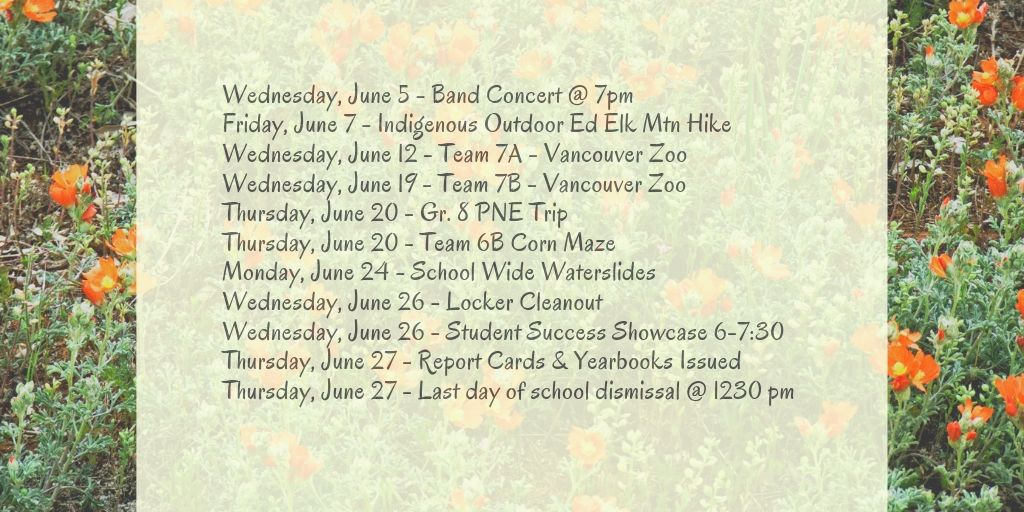March

• Mar. 4th - Quiz (equations); Two Step Equations
• Mar. 7th - Two Step Equations; Word Problems
• Mar. 8th - Quiz (two step equations); Word Problems; Review
• Mar. 11th - Review
• March 13th - Two-Step Equations TEST
• March 14th - π Pi Day! π

February

• Feb. 1st - Subtracting Integers - Textbook # 6-30, 35 - Due Monday Feb. 4th
• Feb. 4th - Dividing Integers (Multiplying Rules Review) - Due Friday Feb. 6th
• Feb. 6th - Order of Operations with Integers - Due Friday Feb 8th at end of class
• Feb. 8th - Quiz; Review/Work Block
• Feb. 11th - Review | Mark Review
• Feb. 15th - UNIT TEST
• Feb. 19th - Variables in Expressions: Textbook p. 197 #1-33, odds Due Thurs. Feb 21st
• Feb. 20th - Words and Symbols: p.199 #1-8, 18-25 Due Thurs. Feb 21st
• Feb. 21st/25th - Solving Equations: Textbook p.201 #1-35, odds Due Wed. Feb 27th
• Feb. 27th - Quiz; Equations whiteboard practice class

January

• Jan. 7th - Percent of a number: mental methods 1% and 10%; Class Activity and Notes
• Jan. 9th - 1-10% of a Number Worksheet - due Friday 11th
• Jan. 11th - Sales and Tax Worksheet - due Monday 14th
• Jan. 14th - Start of Sales Shopping Project --> buying your 10 gifts (Step 1 and 2)
• Jan. 16th - Project: Caluclating sale prices; total amount saved (Step 2) completed
• Jan. 18th - Project: Caluclating sale prices; making \$200 step 3. PRICE LIST BELOW

PROJECT DUE MONDAY JAN. 21ST

• Jan. 21st - Intro to Integers: Opposites and Ordering; front side of worksheet

due Wed. Jan. 23rd

• Jan. 23rd - Number Lines and Adding Integers - front side only - due Friday 25th
• Jan. 25th - Subtracting Integers - back side - due Monday Jan. 28th
• Jan. 28th - QUIZ - Adding and Subtracting Integers
• Jan. 30th - Multiplying Integers - Worksheet Due Friday Feb. 1st

December

• Dec. 5th - Decimals <--> Percents ~ Marked in Class
• Dec. 7th - Fractions Review
• Dec. 10th - Decimals/Percents Quiz; Fractions --> Decimals
• Dec. 12th - Decimals --> Fractions; Worksheet due Friday Dec. 13th
• Dec. 14th - Fractions, Decimals, and Percentages Chart and Word Problems
• Dec. 19th - Unit End => Review and "Big Quiz"

Information & AnnouncementsSchool Bus Registration Opens May 1st!NewsEventsRecent Tweets